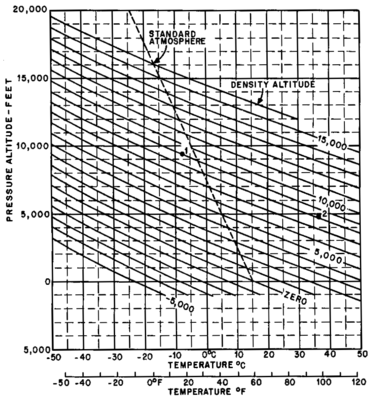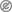# Density altitude

Density altitudeDensity Altitude Computation Chart.

Density altitude is the altitude in the International Standard Atmosphere at which the air density would be equal to the actual air density at the place of observation, or, in other words, the height when measured in terms of the density of the air rather than the distance from the ground. "Density Altitude" is the pressure altitude adjusted for non-standard temperature.

Both an increase in temperature and, to a much lesser degree, humidity will cause an increase in density altitude. Thus, in hot and humid conditions, the density altitude at a particular location may be significantly higher than the true altitude.

## Aircraft safety

Air density is perhaps the single most important factor affecting aircraft performance. It has a direct bearing on:

• The lift generated by the wings — reduction in air density reduces the wing's lift.
• The efficiency of the propeller or rotor — which for a propeller (effectively an airfoil) behaves similarly to lift on wings.
• The power output of the engine — power output depends on oxygen intake, so the engine output is reduced as the equivalent "dry air" density decreases and produces even less power as moisture displaces oxygen in more humid conditions.

Aircraft taking off from a "hot and high" airport such as the Quito Airport or Mexico City are at a significant aerodynamic disadvantage. The following effects result from a density altitude which is higher than the actual physical altitude:

• The aircraft will accelerate slower on takeoff as a result of reduced power production.
• The aircraft will need to achieve a higher true airspeed to attain the same lift - this implies both a longer takeoff roll and a higher true airspeed which must be maintained when airborne to avoid stalling.
• The aircraft will climb slower as the result of reduced power production and lift.

Due to these performance issues, a plane's takeoff weight may need to be lowered or takeoffs may need to be scheduled for cooler times of the day. Wind direction and runway slope may need to be taken into account.

## Calculation

Density altitude can be calculated from atmospheric pressure and temperature (assuming dry air).$\mathrm{DA} = 145442.156 \left[1-\left(\frac{P/P_{SL}}{\mathrm{T}/T_{SL}}\right)^b\right]$

where

DA = density altitude in feet
P = atmospheric (static) pressure
PSL = standard sea level atmospheric pressure (1013.25 hPa ISA or 29.92126 US))
T = true (static) air temperature in kelvins (K) [add 273.15 to the Celsius (°C)] figure
TSL = ISA standard sea level air temperature in kelvins (K) (288.15 K)
b = 0.234969

Note that the leading coefficient is derived from the ratio of the ISA sea level temperature (288.15 K) to ISA Temperature Lapse Rate (6.5 K/km), with the product converted into feet.

National Weather Service Equation

The National Weather Service uses the following dry-air approximation of the above equation in their standards.$\mathrm{DA} = 145442.16 \left[1-\left(\frac{17.326 P}{459.67+T}\right)^{0.235}\right]$

where

DA = density altitude in feet
P = Is the station pressure (atmospheric static pressure) in inches of mercury (inHg)
T = T is the station temperature (atmospheric temperature) in Fahrenheit (F)

Note that the NWS standard specifies that the density altitude should be rounded to the nearest 100 feet.

Easy formula to calculate density altitude from pressure altitude

This is an easier formula to calculate (with great approximation) density altitude from pressure altitude ..and International Standard Atmosphere temperature deviation

Density altitude in feet = pressure altitude in feet + (120 x (OAT - ISA_temperature))

Where:

OAT = Outside air temperature in °C
ISA_temperature = 15 °C - (1.98 °C/1000 ft × actual altitude in thousands of feet)

## References and notes

1. ^ a b AOPA Flight Training, Volume 19, Number 4; April 2007; Aircraft Owners and Pilots Association; ISSN 1047-6415This article incorporates public domain material from the United States Government document "Pilot's Handbook of Aeronautical Knowledge".

Wikimedia Foundation. 2010.

Нужно решить контрольную?

### Look at other dictionaries:

• density altitude — An atmospheric density expressed in terms of the altitude, which corresponds with that density in the standard atmosphere. It is the pressure altitude corrected for temperature. Density altitude is used for computing and predicting aircraft and… …   Aviation dictionary

• density altitude — atmosferos tankio aukštis statusas T sritis Gynyba apibrėžtis Atmosferos tankis, išreikštas aukščiu ir atitinkantis standartinį oro slėgį. atitikmenys: angl. density altitude pranc. densité altitude …   NATO terminų aiškinamasis žodynas

• density altitude — An atmospheric density expressed in terms of the altitude which corresponds with that density in the standard atmosphere …   Military dictionary

• density altitude — noun : the altitude that corresponds to a given air density in a standard atmosphere …   Useful english dictionary

• Density of air — The density of air, ρ (Greek: rho) (air density), is the mass per unit volume of Earth s atmosphere, and is a useful value in aeronautics and other sciences. Air density decreases with increasing altitude, as does air pressure. It also changes… …   Wikipedia

• Altitude — For other uses, see Altitude (disambiguation). Not to be confused with Attitude. Altitude or height is defined based on the context in which it is used (aviation, geometry, geographical survey, sport, and more). As a general definition, altitude… …   Wikipedia

• altitude — i. The vertical distance of a level, a point, or an object considered as a point, measured from mean sea level (ICAO). In the case of aircraft in flight, it is normally expressed in flight levels or hundreds of feet. For example, an aircraft… …   Aviation dictionary

• altitude — The vertical distance of a level, a point or an object considered as a point, measured from mean sea level. See also density altitude; drop altitude; elevation; minimum safe altitude; pressure altitude; transition altitude; true altitude …   Military dictionary

• Altitude diving — is scuba diving where the surface is 300 meters (1,000 feet) or more above sea level (for example, a mountain lake). [cite book |title=Encyclopedia of Recreational Diving, 3rd edition |last=Brylske |first=A. |year=2006 |publisher=PADI… …   Wikipedia

• Altitude sickness — Infobox Disease Name = Altitude sickness Caption = DiseasesDB = 8375 DiseasesDB mult = DiseasesDB2|29615 ICD10 = ICD10|T|70|2|t|66 ICD9 = ICD9|E902.0 ICDO = OMIM = MedlinePlus = eMedicineSubj = med eMedicineTopic = 3225 MeshID = D000532 Altitude… …   Wikipedia